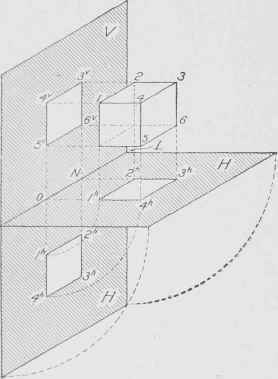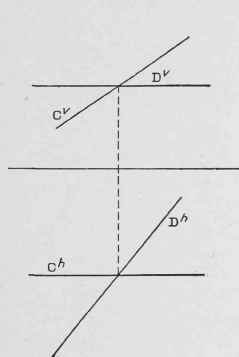Principles. Orthographic projection is the art of representing objects by casting their outlines upon two planes at right angles to each other, so that from the outlines alone the forms and positions of the objects may be determined. The planes of projection are . pictured in Fig. 95, one plane, H, being horizontal, and the other, V, vertical. The intersections of these planes are a straight line GL, known as the ground line.

Orthographic projection requires two distinct processes of the imagination; first, to conceive each view of the corresponding plane of projection; second, to find the position of the different views with respect to each other and to the ground line when the planes of projection have been made into one plane, Fig. 96. A draftsman becomes so accustomed to this process that he is not conscious of any action of the imagination, but pictures the different views of an object without thinking of the planes of projection. The ability to obtain adequate mental pictures of the horizontal and vertical projections of an object - called flan and elevation, respectively-must therefore be acquired by practice.

Plan and Elevation. To illustrate a case. Suppose a one-inch cube to be placed, as shown in Fig. 95, with the top face horizontal and the front face parallel to the vertical plane. Now, suppose the sun is in such a position that its rays are perpendicular to the horizontal, or H, plane, then a shadow of the cube will be cast or projected on the II plane; this shadow called the plan, will be a one-inch square, 1h-2h-3h-4h, Fig. 95.

Copyright, 1911, by American School of Correspondence.

If the sun is in a position such that its rays are perpendicular to the V plane a similar square 3-4-5-6, Fig. 95, will be projected on this plane as the elevation. In order that the projected views, or projections, Fig. 96, as they are better known, may be shown on one plane or on a sheet of drawing paper, the plane H with the projection upon it is revolved about GL as an axis until it lies in the same plane as V.

Fig. 95 represents the picture and is for the purpose of showing the student how the object is projected to the horizontal and vertical planes. Fig. 96 represents the actual projections, plan and elevation, as the problem would be drawn. The projections of a point a and line B drawn according to the first method are shown in Fig. 97.Fig;. 95. Object and Its Projections.Fig. 96. Vertical and Horizontal Projections.

It may be seen from the above explanation that the projected view of a point of an object on a plane is in a perpendicular drawn to the plane through the point of the object.

In Fig. 95, the lines 1h O and 2h N, being perpendicular to GL, are in the same straight lines as 5v O and 6" N, which also are perpendicular to GL. That is - two views of a point are always in aline perpendicular to GL. From this it is evident that the plan must be vertically below the elevation, point for point. Now looking directly at the two planes in the revolved position, a true orthographic projection of the cube is obtained, as shown in Fig. 96.

All points on an object at the same height must appear in elevation at the same distance above the ground line. If numbers 1, 2, 3, and 4 on the plan, Fig. 96, indicate the top corners of the cube, then these four points, being at the same height, must be shown in elevation at the same height and at the top, 4v/1v and 3v/2v From the same figure it is seen that the top of the cube, 1-2-3-4, is shown in elevation as the straight line 4v/1v and 3v/2v. This illustrates the fact that if a surface is perpendicular to either plane of projection, its projection on that plane is simply a line - a straight line if the surface is plane, a curved line if the surface is curved. From Fig. 96 it is seen that the top edge of the cube, 1-4, has for its projection on the vertical plane the point 4v/1v the principle of which is stated in this way: If a straight line is perpendicular to either V or H, its projection on that plane is a point, and on the other plane is a line equal in length to the line itself, and perpendicular to the ground line.Fig. 97. Projections of a Point and a Line.Fig. 98. Typical Projections.

Typical Examples. Fig. 98 is given as an exercise to show clearly the idea of plan and elevation.

.4 = a point B" above H, and A" in front of V

B = square prism resting on H, two of its faces parallel to V

C = circular disk in space parallel to V

D = triangular card in space parallel to V

E = cone resting on its base on H

F = cylinder perpendicular to V, and with one end resting against V

G = line perpendicular to H

H = triangular pyramid above H, with its base resting against V

Suppose in Fig. 99, that it is desired to construct the projections of a prism 1 1/2 in. square, and 2 in. long, standing on one end on the horizontal plane, two of its faces being parallel to the vertical plane. In the first place, as the top end of the prism is a square, the top view or plan will be a square of the same size, that is, 1 1/2 in. Then, since the prism is placed parallel to and in front of the vertical plane, the plan, 1 1/2 in. square, will have two edges parallel to the ground line. As the front face of the prism is parallel to the vertical plane its projection on V will be a rectangle, equal in length and width to the length and width respectively of the prism, and as the prism stands with its base on H, the elevation, showing height above H, must have its base on the ground line. Observe carefully that points in elevation are vertically over corresponding points in plan.

The second drawing in Fig. 99 represents a prism of the same size as the first but lying on one side on the horizontal plane, and with the ends parallel to V.

Summary. The principles which have been used thus far may be stated as follows:

1. If a line or point is on either plane, its other projection must be in the ground line.

2. Height above // is shown in elevation as height above the ground line, and distance in front of the vertical plane is shown in plan as distance below the ground line.Fig. 99. Projections of a Square Prism.

3. If a line is parallel to either plane, its actual length is shown on that plane, and its other projection is parallel to the ground line. A line oblique to either plane has its projection on that plane shorter than the line itself, and its other projection oblique to the ground line. No projection can be longer than the line itself. If two lines intersect, their projections must cross, since the point of intersection of the lines is a point on both lines, and therefore the projections of this point must be on the projections of both lines, or at their intersection. In order that intersecting lines may be represented, the vertical projections must intersect in a point vertically above the intersection of the horizontal projections. Thus Fig. 100 represents two lines which do intersect as Cv crosses Dv at a point vertically above the intersection of Ch and Dh. In Fig. 101, however, the lines do not intersect since the intersections of their projections do not lie in the same vertical line.

4. A plane surface if parallel to either plane, is shown on that plane in its true size and shape; if oblique it is shown smaller than the true size, and if perpendicular it is shown as a straight line. Lines parallel in space must have their V projections parallel to each other and also their H projections.Fig. 100. Projections of Two Intersecting Lines.Fig. 101. Projections of Two Non-Intersecting Lines.